# CCS deployment by source and cumulative carbon dioxide stored (Figure 2.17)¶

## Notebook sr15_2.4.2.3_ccs_deployment¶

This notebook is based on the Release 1.1 of the IAMC 1.5C Scenario Explorer and Data and refers to the published version of the IPCC Special Report on Global Warming of 1.5C (SR15).

The notebook is run with pyam release 0.5.0.

The source code of this notebook is available on GitHub (release 2.0.2).

sr15_2.4.2.3_ccs_deployment

### IPCC SR15 scenario assessment¶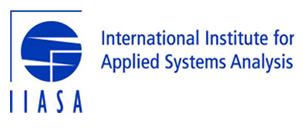# Analysis of carbon capture and sequestration (CCS)¶

This notebook computes indicators and diagnostics of the deployment of CCS by fuel and the total amount of CO2 stored as shown in Figure 2.17 in the IPCC's "Special Report on Global Warming of 1.5°C".

The scenario data used in this analysis can be accessed and downloaded at https://data.ene.iiasa.ac.at/iamc-1.5c-explorer.

## Load pyam package and other dependencies¶

In :
import pandas as pd
import numpy as np
import io
import itertools
import yaml
import math
import matplotlib.pyplot as plt
%matplotlib inline
import pyam

from utils import boxplot_by_cat

pyam - INFO: Running in a notebook, setting pyam logging level to logging.INFO and adding stderr handler


## Import scenario data, categorization and specifications files¶

Alternatively, it can be re-created using the notebook sr15_2.0_categories_indicators.

The last cell of this section loads and assigns a number of auxiliary lists as defined in the categorization notebook.

In :
sr1p5 = pyam.IamDataFrame(data='../data/iamc15_scenario_data_world_r2.0.xlsx')

pyam.utils - INFO: Reading ../data/iamc15_scenario_data_world_r2.0.xlsx

In :
sr1p5.load_meta('sr15_metadata_indicators.xlsx')

pyam.core - INFO: Importing metadata for 416 scenarios (for total of 416)

In :
with open("sr15_specs.yaml", 'r') as stream:

rc = pyam.run_control()
for item in specs.pop('run_control').items():
rc.update({item: item})
cats = specs.pop('cats')
all_cats = specs.pop('all_cats')
subcats = specs.pop('subcats')
all_subcats = specs.pop('all_subcats')
plotting_args = specs.pop('plotting_args')
marker= specs.pop('marker')


## Downselect scenario ensemble to categories of interest for this assessment¶

Use all years (as of 2020) to correctly compute the total amount of CO2 stored in each scenario.

In :
years = range(2020, 2101, 5)

In :
cats.remove('Above 2C')

In :
df = sr1p5.filter(category=cats, year=years)


## Set specifications for filter and plotting and initialize a data list¶

In :
plot_ylabel = '{} with CCS (EJ)'
save_name = 'output/fig2.17{}.{}'
figure_format = 'png'

In :
filter_args = dict(df=sr1p5, category=cats, marker=None, join_meta=True)

In :
def plotting_args(name, panel_label=None, filetype=figure_format):
return {'categories': cats, 'column': 'category', 'years': range(2020, 2101, 10), 'add_marker': marker,
'ylabel': plot_ylabel.format(name),
'save': save_name.format(name if panel_label is None else '{}_{}'.format(panel_label, name), filetype)}

In :
data = []


## Add IEA's 'Faster Transition Scenario' to the set of marker scenarios for comparison¶

In :
m = 'IEA WEM'
col = 'marker'
sr1p5.set_meta(m, col,
sr1p5.filter(model='IEA World Energy Model 2017',
scenario='Faster Transition Scenario'))
rc.update({'marker': {col: {m: 'o'}},
'c': {col: {m: 'red'}},
'edgecolors': {col: {m: 'black'}}}
)
marker += [m]


## Extract CCS timeseries data by fuel¶

In :
ccs_bio = (
pyam.filter_by_meta(
df.filter(variable='Primary Energy|Biomass|Modern|w/ CCS')
.timeseries(), **filter_args)
)

In :
name = 'bioenergy'
fig = boxplot_by_cat(ccs_bio, **plotting_args(name, 'a'))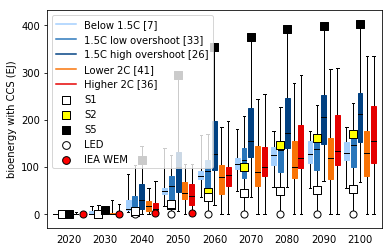In :
data.append(('Bioenergy with CCS', ccs_bio))

In :
ccs_coal = (
pyam.filter_by_meta(
df.filter(variable='Primary Energy|Coal|w/ CCS')
.timeseries(), **filter_args)
)

In :
name = 'coal'
boxplot_by_cat(ccs_coal, **plotting_args(name, 'b'), legend=False)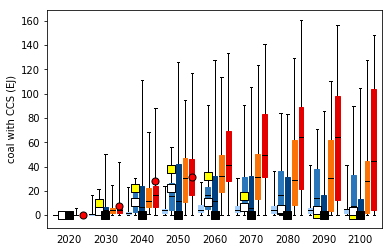In :
data.append(('Coal with CCS', ccs_coal))

In :
ccs_gas = (
pyam.filter_by_meta(
df.filter(variable='Primary Energy|Gas|w/ CCS')
.timeseries(), **filter_args)
)

In :
name = 'gas'
boxplot_by_cat(ccs_gas, **plotting_args(name, 'c'), legend=False)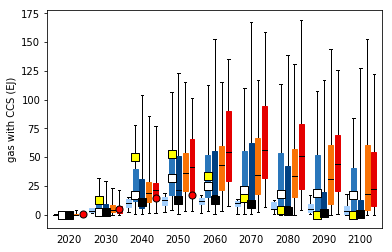In :
data.append(('Gas with CCS', ccs_gas))


## Compute cumulative CO2 stored¶

In :
ccs = (
df.filter(variable='Carbon Sequestration|CCS')
.convert_unit('Mt CO2/yr', 'Gt CO2/yr')
.timeseries()
)
ccs.index = ccs.index.droplevel([2, 3, 4])

In :
cum_ccs = pd.DataFrame()
for i in range(2030, 2100, 10):
cum_ccs[i] = ccs.apply(pyam.cumulative, raw=False, axis=1, first_year=2020, last_year=i)

cum_ccs = pyam.filter_by_meta(cum_ccs, **filter_args)

In :
boxplot_by_cat(
cum_ccs,
categories=cats, column='category', years=range(2030, 2100, 10),
ylabel='cumulative CO2 stored (Gt)',
save=save_name.format('d_cumulative_ccs', figure_format),

/Users/dh/anaconda3/lib/python3.7/site-packages/matplotlib/axes/_base.py:3610: MatplotlibDeprecationWarning:
The ymax argument was deprecated in Matplotlib 3.0 and will be removed in 3.2. Use top instead.
alternative='top', obj_type='argument')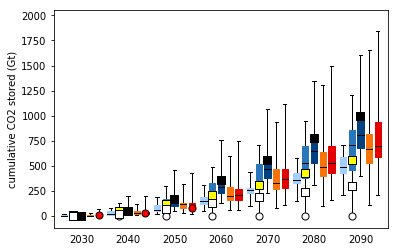In :
data.append(('Cumulative CCS', cum_ccs))


## Export timeseries data to xlsx¶

In :
writer = pd.ExcelWriter('output/fig2.17_data_table.xlsx')
for (name, _df) in data:
pyam.utils.write_sheet(writer, name, _df, index=True)
writer.save()

In [ ]: